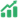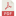# Pengaruh Model PjBL dan Motivasi Belajar Terhadap Hasil Belajar Mapel DDTK Kelas X TITL SMKN 1 Sidoarjo

## Authors

• Rifki Firmansyah Universitas Negeri Surabaya
• Meini Sondang Sumbawati Universitas Negeri Surabaya

## Abstract

Kata Kunci: Project Based Learning, Direct Instruction, Motivasi Belajar, Hasil Belajar.

In carrying out teaching and learning activities, of course, a learning model is needed that can support student learning. In the Implementation of the Independent Curriculum (IKM), the learning model project based learning (PjBL) is one of the recommended learning models to be applied to the independent curriculum. This study aims to: (1) determine the effect of PjBL and DI learning models on student learning outcomes; (2) Knowing the effect of student learning outcomes with high and low learning motivation; (3) Knowing the interaction of PjBL and DI learning models on learning outcomes in terms of student learning motivation. The research was conducted on the subject of the basics of electrical engineering class X TITL SMKN 1 Sidoarjo with a focus on elements of electrical engineering drawing software. The research method used is quasi-experimental with a 2×2 factorial design. The subjects of this study were class X TITL 1 as the experimental class and class X TITL 2 as the control class at SMKN 1 Sidoarjo. Then to analyze the data used the normality test, homogeneity test, and two-way ANOVA test. The results showed that: (1) There was an influence of the PjBL and DI learning models on cognitive and psychomotor learning outcomes with significant values of 0.000 and 0.000. However, there was no effect on psychomotor learning outcomes with a significance value of 0.965; (2) There is an effect of affective and psychomotor learning outcomes on students who have high and low motivation to learn with a significance value of 0.000 and 0.000. However, there is no effect on cognitive learning outcomes with a significance value of 0.140; (3) There is an interaction between the PjBL and DI learning models on cognitive learning outcomes in terms of student learning motivation with a significance value of 0.034. However, there was no interaction with affective and psychomotor learning outcomes with significance values of 0.812 and 0.695 obtained.

Keywords: Project Based Learning, Direct Instruction, Learning Motivation, Learning Outcomes.Abstract views: 186 ,PDF Downloads: 143